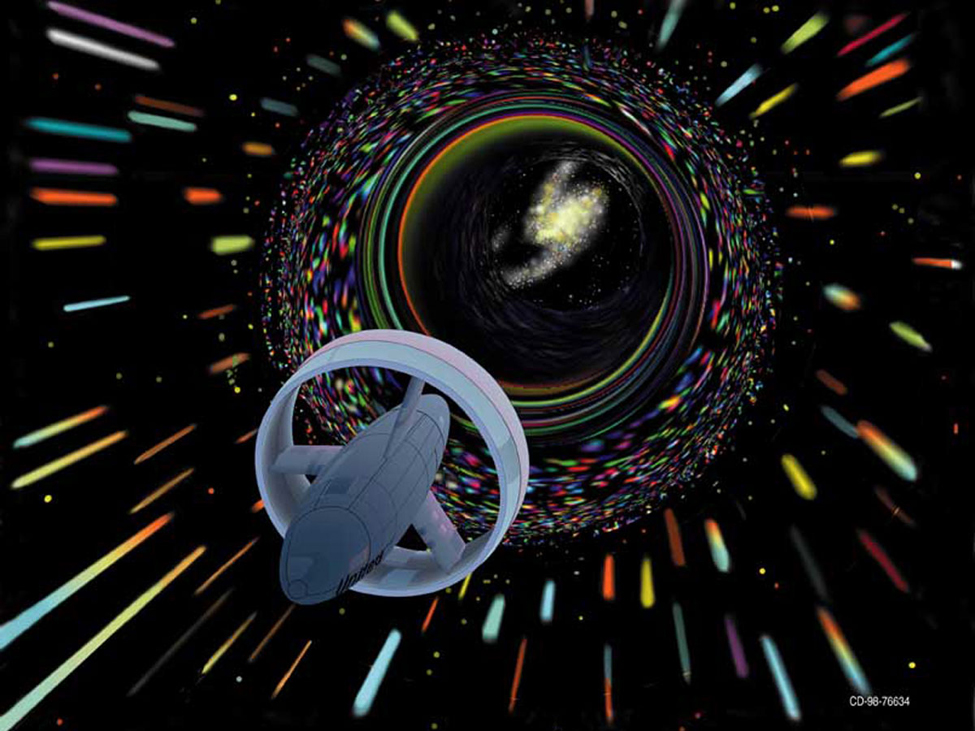In Progress
Lesson 8 of 24
In Progress

# IntroductionFigure 1. The vision of human exploration by the National Aeronautics and Space Administration (NASA) to distant parts of the universe illustrates the idea of space travel at high speeds. But, is there a limit to how fast a spacecraft can go? (credit: NASA)

Science fiction writers often imagine spaceships that can travel to far-off planets in distant galaxies. However, back in 1905, Albert Einstein showed that a limit exists to how fast any object can travel. The problem is that the faster an object moves, the more mass it attains (in the form of energy), according to the equation,

whereis the object’s mass at rest,is its speed, andis the speed of light. What is this speed limit? (We explore this problem further in (Figure).)

The idea of a limit is central to all of calculus. We begin this chapter by examining why limits are so important. Then, we go on to describe how to find the limit of a function at a given point. Not all functions have limits at all points, and we discuss what this means and how we can tell if a function does or does not have a limit at a particular value. This chapter has been created in an informal, intuitive fashion, but this is not always enough if we need to prove a mathematical statement involving limits. The last section of this chapter presents the more precise definition of a limit and shows how to prove whether a function has a limit.